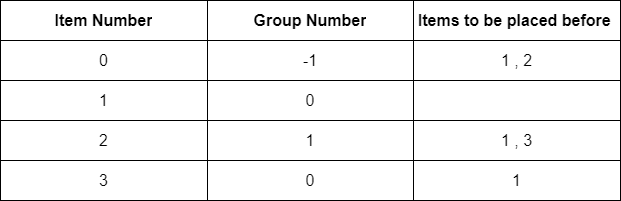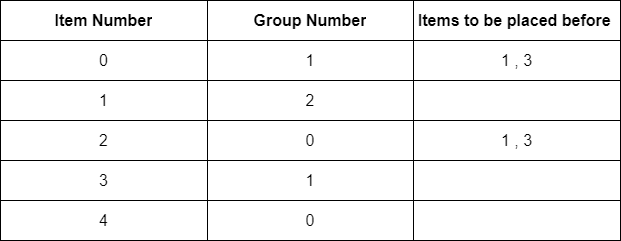0

Sort Items By Group

Difficulty: MEDIUM
Avg. time to solve
20 min
Success Rate
85%

Problem Statement

Your task is to sort these items in an order which follows the following rules :

The items belonging to the same group must be ordered adjacent to each other.
The item which does not belong to any group can be placed anywhere.
Each item appearing in BEFORE[i] must be placed before the ith item in the sorted list.
Input Format :
The first line contains an integer ‘T’, which denotes the number of test cases to be run. Then, the T test cases follow.

The first line of each test case contains two space-separated integers, ‘N’ and ‘M’, denoting the number of items and the number of groups respectively.

The second line of each test case contains ‘N’ space-separated integers, where every ith integer denotes the group to which the ith item belongs. If an item does not belong to any group, “-1” is used at its place.

Then ‘N’ lines follow, and each of these lines contains the following :

An integer ‘X’ denoting the number of items that should be placed before the ith item. Then X space-separated integers follow. Each integer, ‘Y’ denotes the item number that should be placed before the ith item
Output Format :
For each test case, print ‘N’ space-separated integers, denoting the items in the required order.

If there is no order of items that can match all the requirements, print a single integer “-1”.

Output for each test case will be printed in a separate line.
Note :
You do not need to print anything. It has already been taken care of. Just implement the given function.
Constraints :
1 <= T <= 10
1 <= N,M <= 10^5
-1 <= GROUP[I] <= M-1
0 <= X <= N-1
0 <= Y <= N-1

Where ‘GROUP[i]’ is the group to which the ith item belongs.

Time Limit: 1 sec
1
4 2
-1 0 1 0
2 1 2
0
2 1 3
1 1
1 3 2 0
Explanation For Sample Input 1 :In this test case, we can observe that item 0 does not belong to any group while item 1 and item 3 belong to group 0 and item 2 belong to group 1. We also need to consider that item 1 and item 2 should come before item 0. While item 1 and item 3 should come before item 2. While item 1 should come before item 3. We can observe that all these requirements are being met in the given output.
1
5 3
1 2 0 1 0
2 1 3
0
2 1 3
0
0
1 3 0 2 4
Explanation For Sample Input 2 :In this test case, we can observe that item 2 and item 4 belong to group 0 and item 1 belongs to group 2. Item 2 and item 4 belong to group 0. We also need to consider that item 1 and item 3 should come before item 0 and item 2. We can observe that all these requirements are being met in the given output.Console